Repeated integral

(diff) ← Older revision | Latest revision (diff) | Newer revision → (diff)

An integral in which there is a successive integration with respect to different variables, i.e. an integral of the form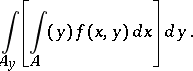(1)

The functionis defined on a setlying in the direct product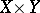of spacesandin which are given-finite measures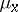andand which have the completeness property; the set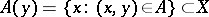(the "section" at "level"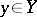of) is measurable with respect to, while the set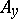(the projection ofon) is measurable with respect to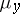. The integration overis performed with respect to, and that overwith respect to. The integral (1) is also denoted by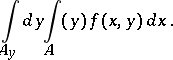Multiple integrals (cf. Multiple integral) can be reduced to repeated integrals.

Let a function, integrable with respect to the measure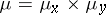on the set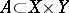, be extended by zero to a function on the entire space. Then the repeated integrals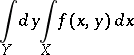and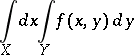exist and are equal to each other: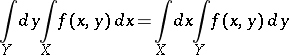(2)

(see Fubini theorem). In the left-hand integral the outer integration is in fact performed over the set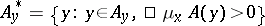. In particular, for points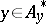the sets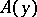are measurable with respect to. In general, one cannot take this integral over the entire setsince, while the set A is measurable with respect to, the setmay be non-measurable with respect to, and similarly, the individual sets,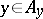, may be non-measurable with respect to. On the other hand, the setis always measurable with respect toprovided only that the setis measurable with respect to.

The above conditions for changing the order of integration in a repeated integral are only sufficient but not necessary; sometimes it is permissible to change the order of integration in a repeated integral while the corresponding multiple integral does not exist. For example, for the function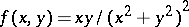for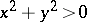and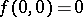the repeated integrals are equal: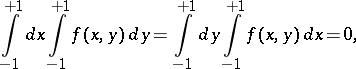while the multiple integraldoes not exist. However, if at least one of the integrals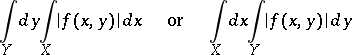is finite, then the functionis integrable on the setand relation (2) holds.

In the case where the inner integral is a Stieltjes integral and the outer one is a Lebesgue integral, the following theorem on changing the order of integration holds: Let a functionbe summable with respect toin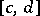for all values ofinand let it be a function of bounded variation with respect toinfor almost-all values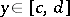. Also, suppose that the total variation ofwith respect to the variableinfor all given values ofdoes not exceed some non-negative summable function on. Then the functionis a function of bounded variation with respect to the variableinand for any continuous functiononone has the formula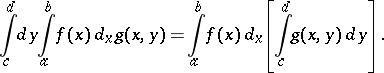How to Cite This Entry:
Repeated integral. Encyclopedia of Mathematics. URL: http://encyclopediaofmath.org/index.php?title=Repeated_integral&oldid=19219
This article was adapted from an original article by L.D. Kudryavtsev (originator), which appeared in Encyclopedia of Mathematics - ISBN 1402006098. See original article